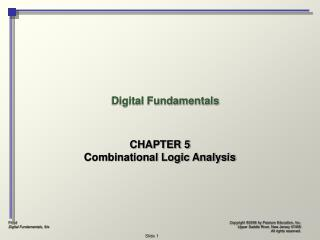# Digital Fundamentals - PowerPoint PPT PresentationDownload PresentationDigital Fundamentals

Digital FundamentalsDownload Presentation## Digital Fundamentals

- - - - - - - - - - - - - - - - - - - - - - - - - - - E N D - - - - - - - - - - - - - - - - - - - - - - - - - - -
##### Presentation Transcript

1. Digital Fundamentals CHAPTER 5 Combinational Logic Analysis

2. Basic Combinational Logic Circuits

3. Basic Combinational Logic Circuits • AND-OR Logic • AND-OR-Invert Logic • Exclusive-OR Logic

4. Basic Combinational Logic Circuits • AND-OR Logic

5. Basic Combinational Logic Circuits • AND-OR Invert Logic

6. Basic Combinational Logic Circuits • Exclusive-OR Logic

7. Implementing Combinational Logic

8. Implementing Combinational Logic • From Boolean expression to logic circuit • Given a Boolean expression, create a logic circuit that implements that expression. • From truth table to logic circuit • Given a truth table, create a logic circuit that implements that table.

9. The Universal Property of NAND and NOR Gates NAND and NOR gates are “universal” because they can used to produce any of the other logic functions.

10. The Universal Property of NAND Gates • NAND Gate as an Inverter

11. The Universal Property of NAND Gates • Two NAND Gates as an AND Gate

12. The Universal Property of NAND Gates • Three NAND Gates as an OR Gate

13. The Universal Property of NAND Gates • Four NAND Gates as OR Gate

14. The Universal Property of NOR Gates • NOR Gate as an Inverter

15. The Universal Property of NOR Gates • Two NOR Gates as an OR Gate

16. The Universal Property of NOR Gates • Three NOR Gates as an AND Gate

17. The Universal Property of NOR Gates • Four NOR Gates as an AND Gate